# Lesson 11 Maximum or Minimum of Quadratic Functions

• Slides: 22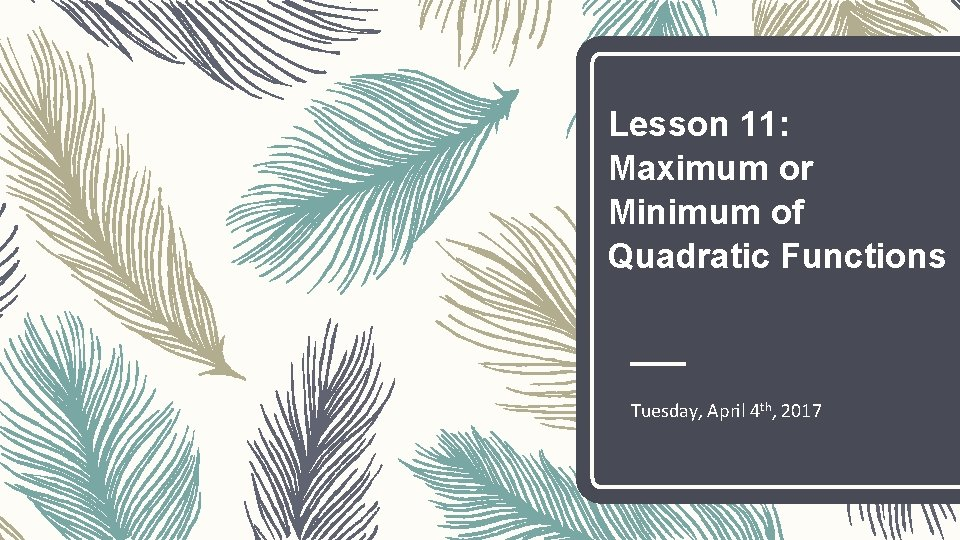Lesson 11: Maximum or Minimum of Quadratic Functions Tuesday, April 4 th, 2017Vertex – The vertex of a parabola is the _______________, also known as the ________ or ________ of a parabola – __________________________________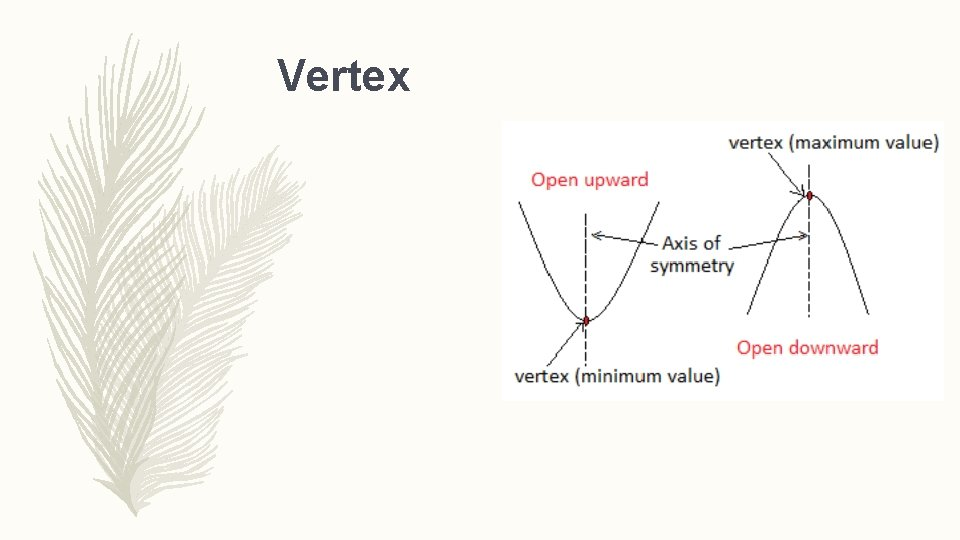Vertex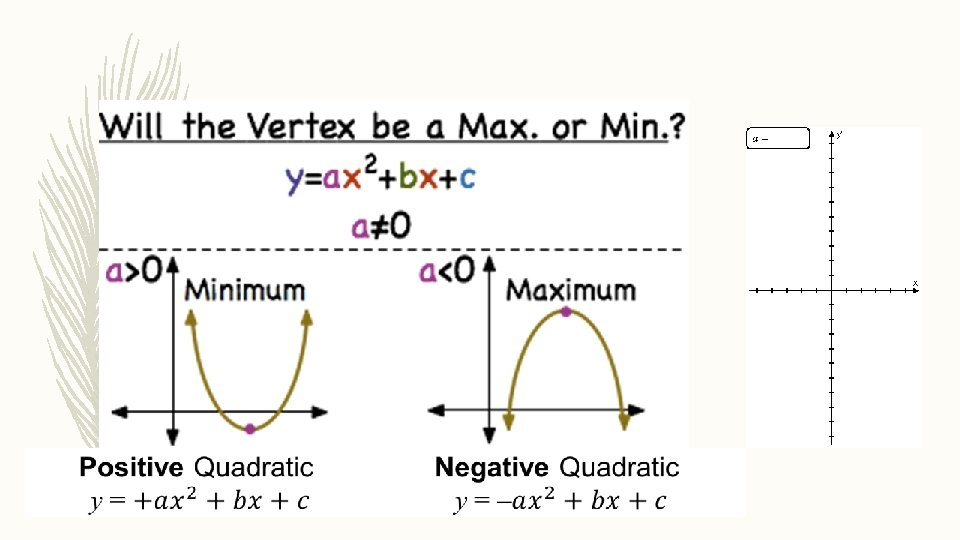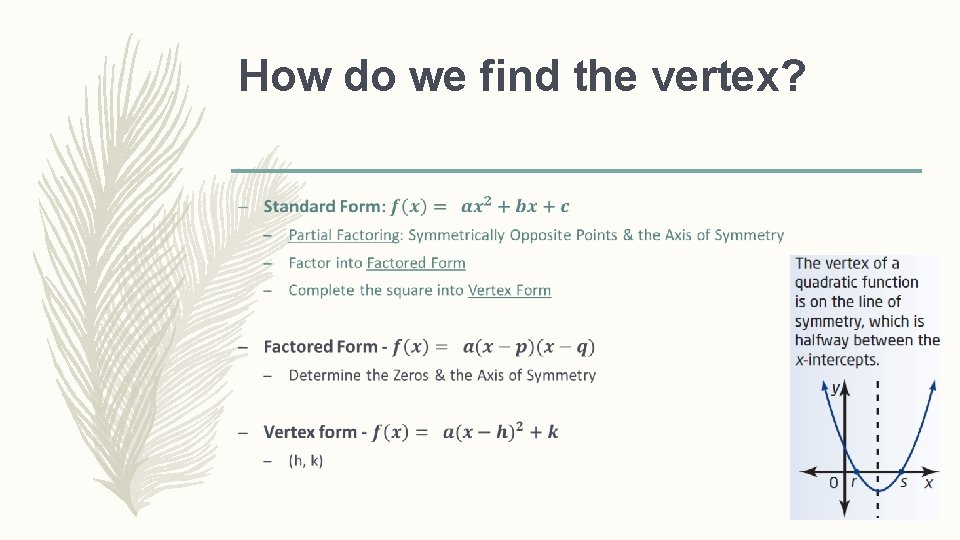How do we find the vertex? –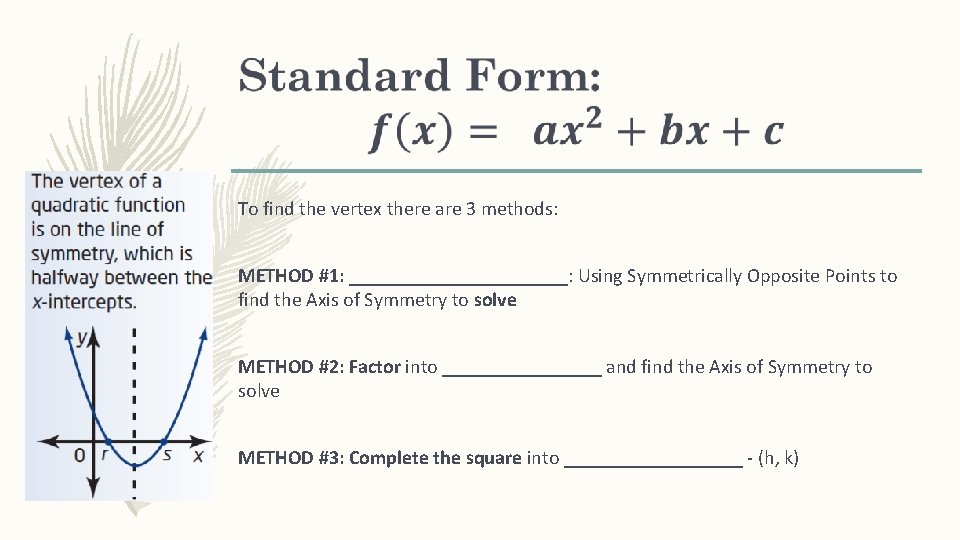To find the vertex there are 3 methods: METHOD #1: ___________: Using Symmetrically Opposite Points to find the Axis of Symmetry to solve METHOD #2: Factor into ________ and find the Axis of Symmetry to solve METHOD #3: Complete the square into _________ - (h, k)Method #1: Partial Factoring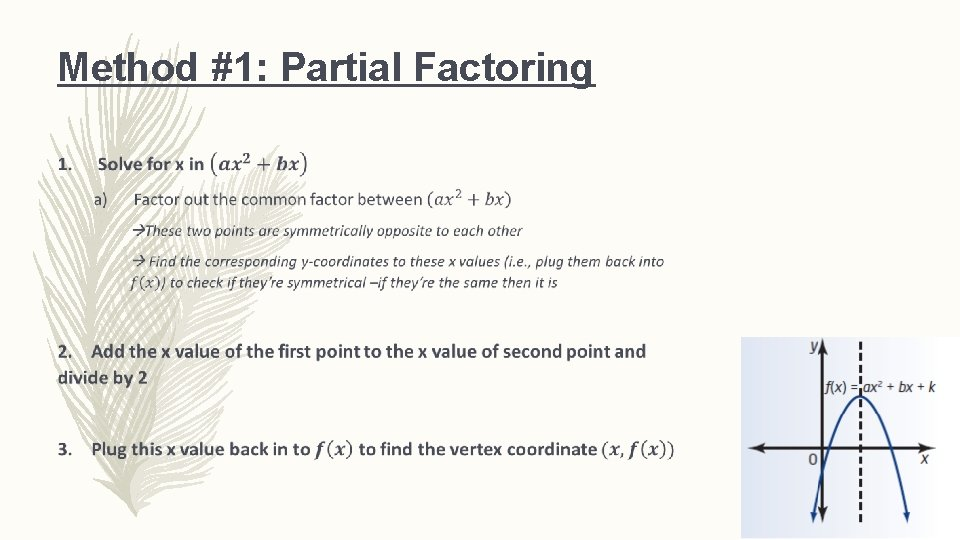Method #1: Partial Factoring –Method #1: Partial Factoring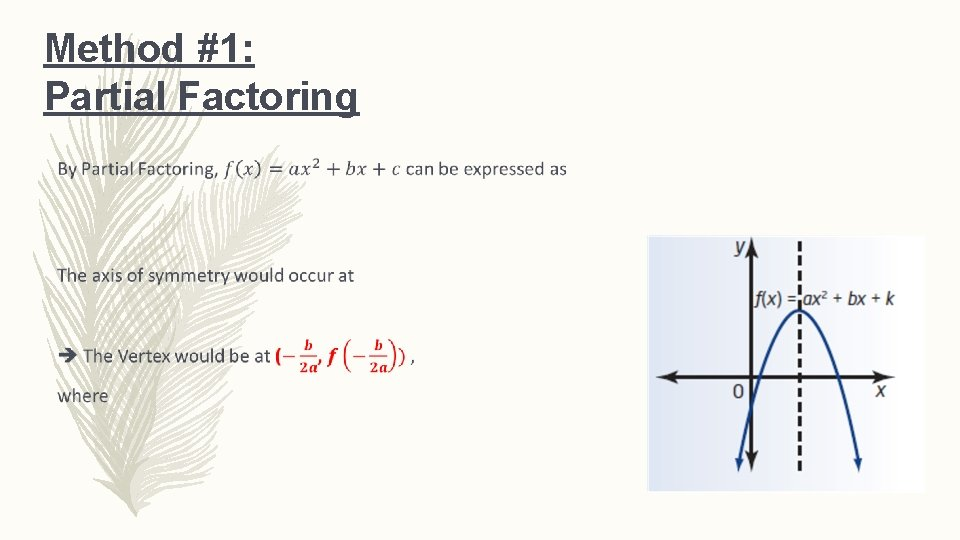Method #1: Partial Factoring –Method #2: Factored Form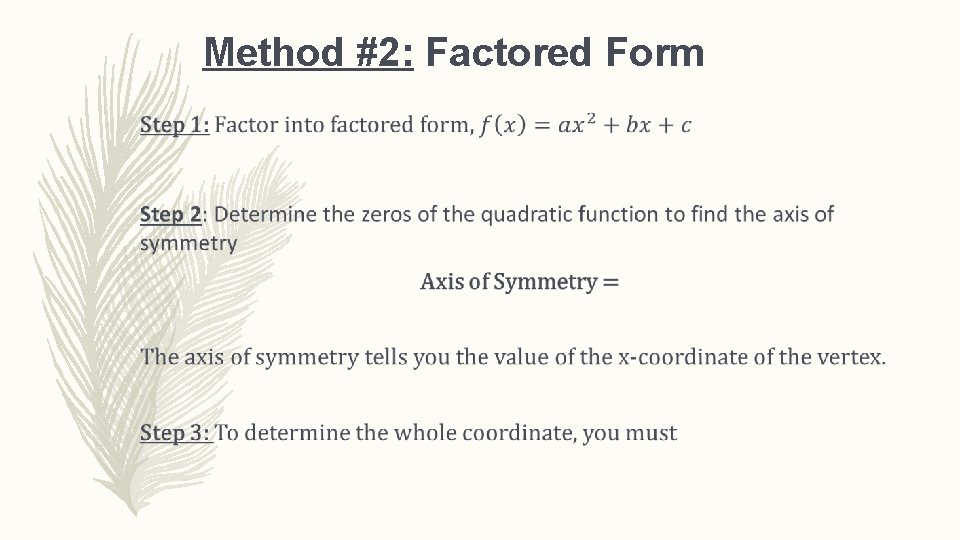Method #2: Factored Form –Method #2: Factor into Factored FormMethod #3: Vertex FormCompleting the Square –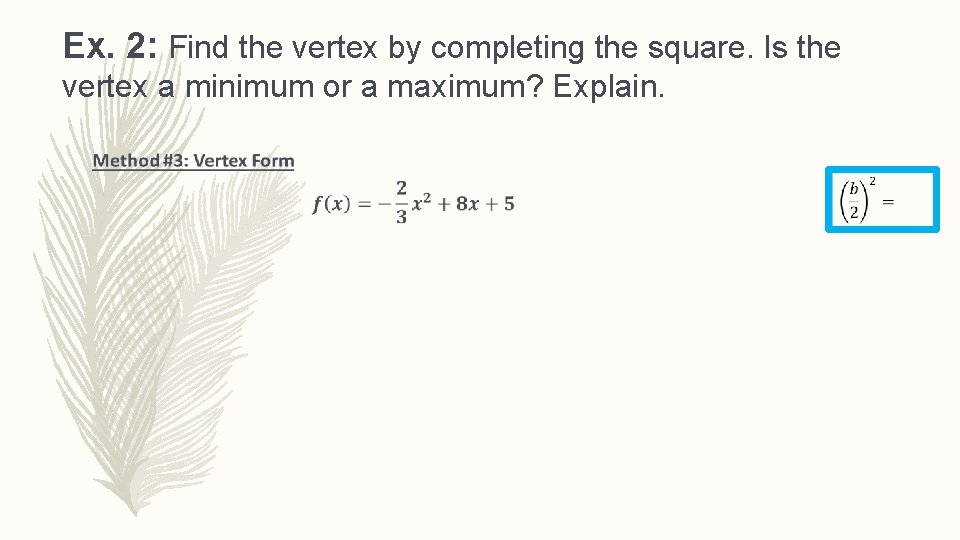Ex. 2: Find the vertex by completing the square. Is the vertex a minimum or a maximum? Explain. –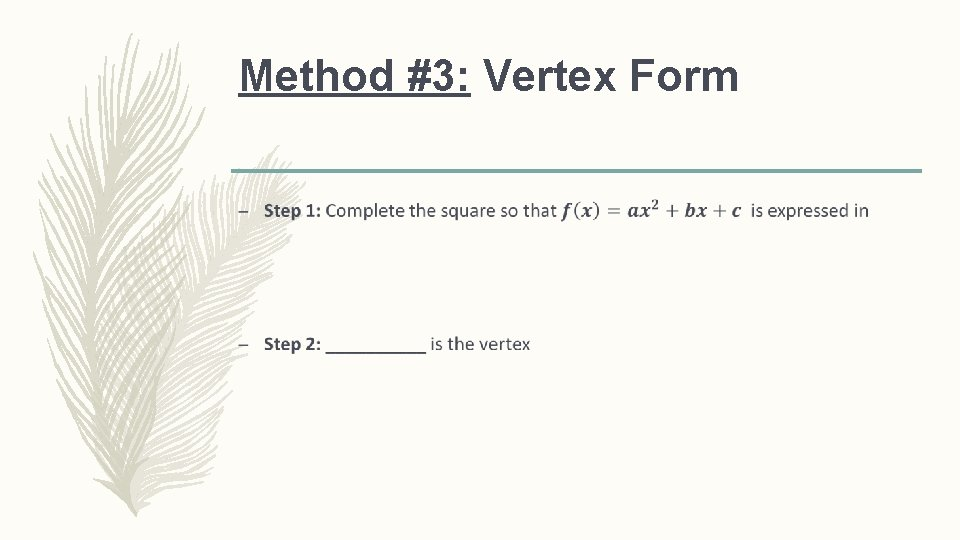Method #3: Vertex Form –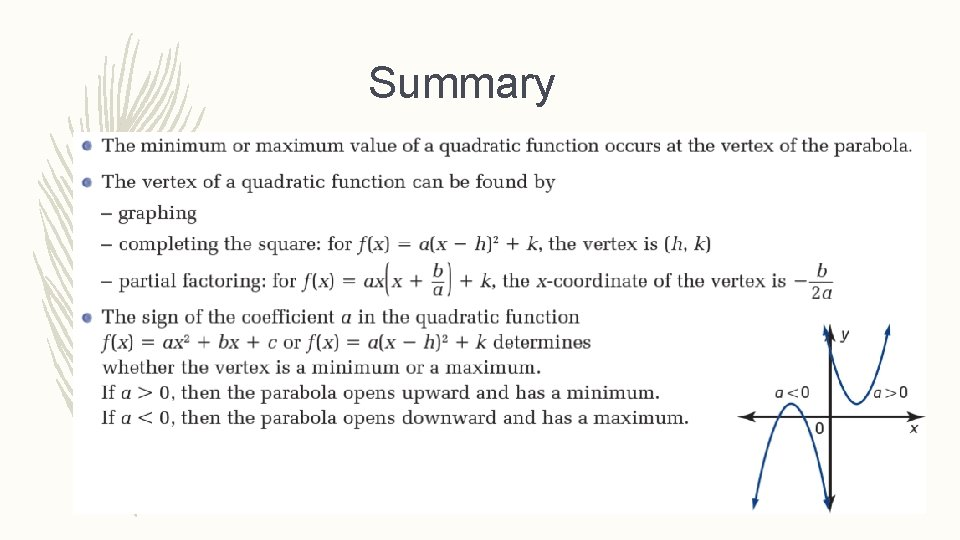Summary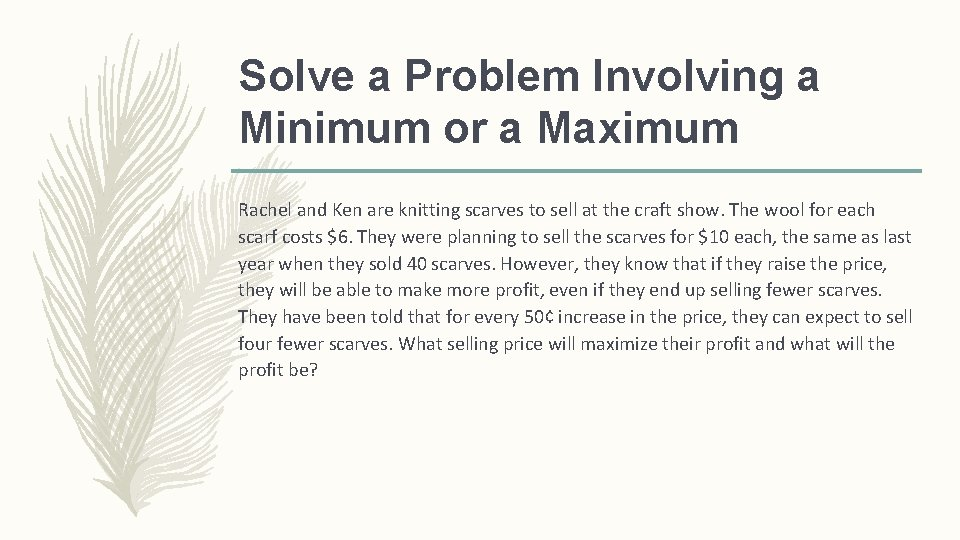Solve a Problem Involving a Minimum or a Maximum Rachel and Ken are knitting scarves to sell at the craft show. The wool for each scarf costs \$6. They were planning to sell the scarves for \$10 each, the same as last year when they sold 40 scarves. However, they know that if they raise the price, they will be able to make more profit, even if they end up selling fewer scarves. They have been told that for every 50¢ increase in the price, they can expect to sell four fewer scarves. What selling price will maximize their profit and what will the profit be?Solve a Problem Involving a Minimum or a Maximum –